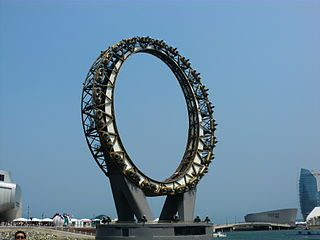Explore BrainMass
Share

# Big-O Notation[Photo Credit: hyolee2]

Big-O notation is used by scientists as a way to denote the time or space complexity of an algorithm as a function of its’ input size. The most common way to denote input size is with the letter n. When determining complexity, big-O notation is helpful as a tool to make the problem simpler. The person analyzing the program will break it down into pieces and get the complexity for each piece, then combine the complexities into a single one for the whole algorithm. Since the notation is in terms of n, any constant coefficient is ignored, so O(2n) is the same as O(n) The most common classes represented in big-O notation, in order from fastest to slowest are:

Constant Time:

O(1) is given to any algorithm or step that runs in the same amount of time regardless of input size. This is the majority of steps in any program, an example is a simple if statement.

Logarithmic Time:

O(logn) denotes logarithmic time. This complexity tends to come up when partitioning sets in half multiple times. The logarithm used is generally base 2.

Linear Time:

O(n) denotes linear time. This tends to come up when doing anything to a dataset as many times as there is data in the set. This arises from having n * O(1) operations, such as in a for loop that loops through an entire set.

Lin-Log Time:

O(nlogn) denotes Lin-Log time. This appears most often in comparison algorithms, where a dataset will be compared to a subset of itself n times, such as sorting algorithms. It also appears across any problems that are reducible to sorting, such as tree balancing, heapify, and convex hull.

Polynomial Time:

O(nk) denotes polynomial time. The most common number for k is 2, where an algorithm will do n operations on each element of the set, such as in a naive comparison sort like bubble sort. Orders above O(n2), and often O(n2) itself are generally considered too slow for use in real time systems if n is larger than a few elements. Linear time is technically a subset of this, where k = 1, but it is significantly faster and so has been given its’ own class by computer scientists.

Exponential Time:

O(2n) denotes exponential time. This is very undesirable, and is generally the result of a brute force algorithm to compute NP-Complete problems. For NP-hard problems, this is often the best solution you can get.

Factorial Time:

O(n!) denotes factorial time. This is very undesirable as for a data set of about 150, it will take longer than the life of the universe to complete the process. An example is the brute force solution to the travelling salesperson problem.